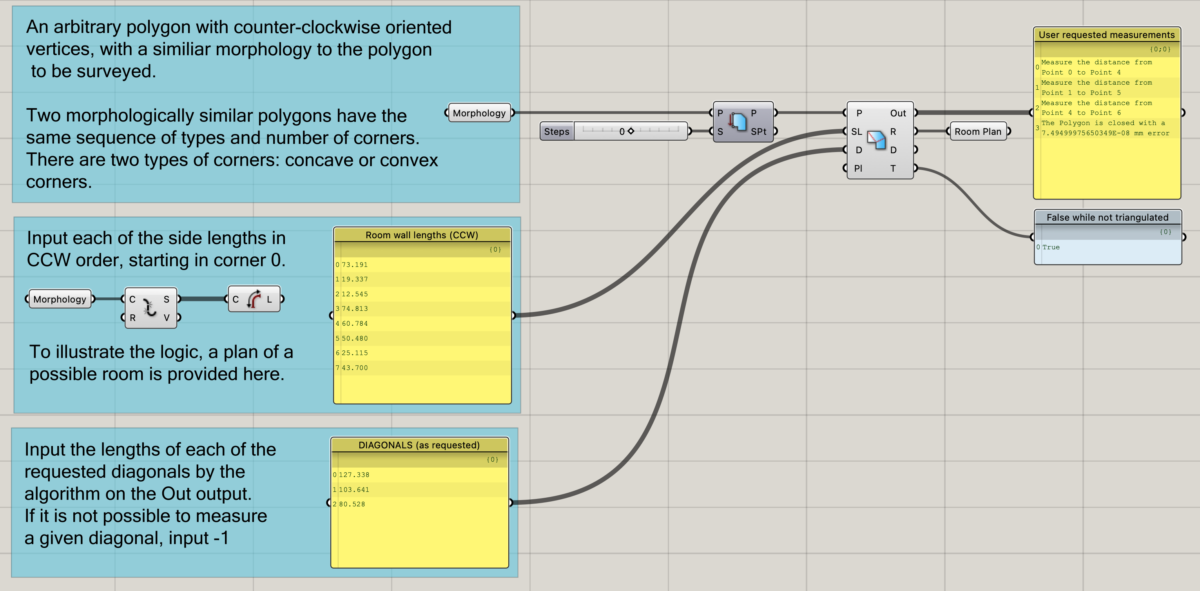# RoomSurvey workflow

The RoomSurvey component is part of the RoomSurveyor plugin and comes in two flavours: RoomSurvey and RoomSurveyStrict. There are two differences between these components: the logic used to request diagonals and the assumption that if a polygonal chain is closed within tolerance the angles are assumed to be correct (which is not always true). The RoomSurvey requests longer diagonals first which is more effective on smaller polygons with few non-orthogonal corners and uses the closed polygon test. You can read more about RoomSurvey here. RoomSurveyStrict makes no assumptions to speed up the process and requests shorter diagonals first. This means that RoomSurveyStrict will always request at least n-3 diagonals, where n is the number of polygon sides. So, for a polygon with 6 sides, 3 diagonals will be requested and for one with 20 sides, 17 diagonals will be required. Choose wisely!

On a conceptual level the survey workflow steps are:

1. Draw or provide a polygon that is similar to the polygon you need to survey
2. Provide the measurements of each of the polygon sides
3. Provide the requested diagonals

### Inputs and Outputs

Both components take the same inputs: a closed polygon (polyline), an ordered list of side lengths and the requested diagonals.  You can input a polygon with any number of sides, convex or concave, with whatever rotation or scaling. The only rule is that the polygon must be morphologically similar to the shape you want to survey. Two polygons are morphologically similar when they have the same number of sides and the same sequence of corner types.

The dimensions of each side must be provided as a counter-clockwise ordered list, starting from the first corner of the polygon. The diagonals are requested by the algorithm in the [out] output. Higher numerical precision of the side and diagonal measurements results in higher accuracy of the reconstruction. For practical purposes, if you are working in meters providing measurements with millimetre precision is a good trade-off.

The component outputs are a list with the user instructions [out], the current reconstruction of the polygon (more on this latter) [R], the requested diagonals as lines [D], and a boolean that informs if the triangulation process has finished [T].

• [out] – Returns instructions to the user for diagonal measurements (e.g.: Measure the distance from Point 3 to Point 5). When the polygon is closed it reports the closing error (e.g.: The Polygon is closed with a 5.18368349574805E-12 mm error)
• [R] – While the polygon is not triangulated it returns the polygonal chain scaled by the lengths of the respective sides with the transformed angles as a result of the provided diagonal measurements. When the process finishes it returns the triangulated polygon, i.e., the surveyed plan or section.
• [D] – The requested diagonals as lines between corners of the provided morphology.
• [T] – A boolean informing if the triangulation process as finished.

### Example Setup

The following image presents the simplest possible setup, with the exception of the ShiftStartPoint component that is only needed to change the starting point of the morphology.